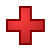Games
Problems
Go Pro!

# Bases

Reference > Mathematics > Number TheoryA simple explanation of bases, using base ten as an exampleUsing Base Eight as a way of introducing bases other than base tenClarification about symbols and notation in basesHow to handle bases that are larger than base ten (for example, base sixteen)How to convert base ten numbers into other basesHow to perform arithmetic operations in bases besides base tenWhat to do if you are not told the base in which a calculation is done?Why do we even use bases other than base ten? What value does it have?Like us on Facebook to get updates about new resources